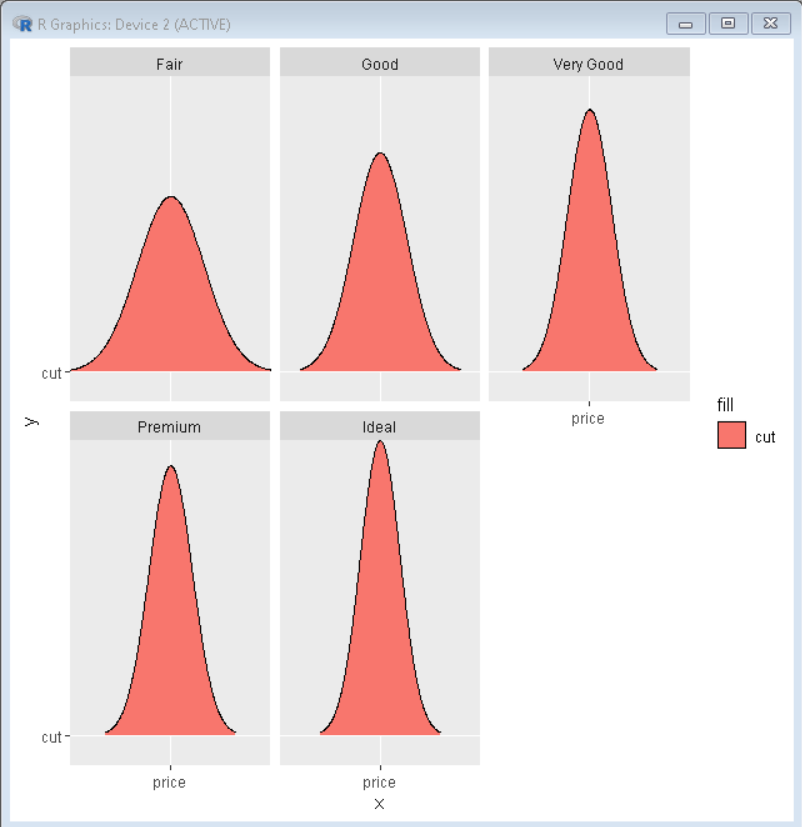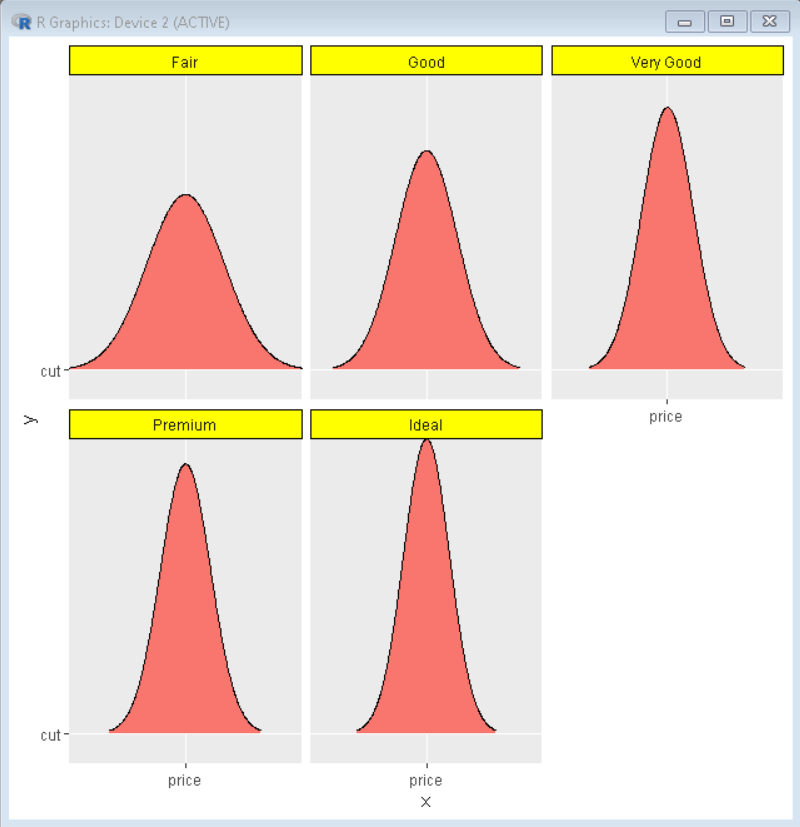Open In App

# How To Change facet_wrap() Box Color in ggplot2 in R?

In this article, we will discuss how to change facet_wrap() box color in ggplot2 in R Programming language.

Facet plots, where one subsets the data based on a categorical variable and makes a series of similar plots with the same scale. Facetting helps us to show the relationship between more than two categories of data. When you have multiple variables, with faceting it can be plotted in a single plot into smaller plots.

We can easily plot a facetted plot using the facet_wrap() function of the ggplot2 package. When we use facet_wrap() in ggplot2, by default it gives a title in a grey box.

Syntax: plot + facet_wrap( ~facet-variable)

Where:

facet-variable: determines the variable around which plots have to be divided.

## Creating a basic facet plot

Here, is a basic facet plot made using the diamonds data frame which is provided by R Language natively. We have used the facet_wrap() function with ~cut to divide the plot into facets according to their cut.

## R

 `# load library ggridges and tidyverse``library``(ggridges)``library``(tidyverse)` `# Basic facet plot divided according to category cut``# diamonds data frame is used in plot which``# is provided natively by R Language``# ggplot() function is used to plot the chart``ggplot``(diamonds, ``aes``(x=``"price"``, y=``"cut"``, fill=``"cut"``)) +` `# geom_density_ridges() function is used to draw ridgeline plot``  ``geom_density_ridges``()+` `# facet_wrap() function divides the plot in facets according to category of cut``  ``facet_wrap``(~cut)`

Output:## Box Color Customization

We can customize various aspects of a ggplot2 using the theme() function. To change the default grey fill color in the facet_wrap() title box, we need to use “strip.background” argument inside the theme() layer with color and fill property of element_rect() function.

Syntax: plot + theme( strip.background = element_rect( colour, fill ))

Where:

• colour: determines the color of outline of box
• fill: determines the color of background fill of box

Example:

In this example, we specified element_rect with yellow fill color and black for box outline color.

## R

 `# load library ggridges and tidyverse``library``(ggridges)``library``(tidyverse)` `# ggplot() function is used to plot the chart``ggplot``(diamonds, ``aes``(x=``"price"``, y=``"cut"``, fill=``"cut"``)) +` `# geom_density_ridges() function is used``# to draw ridgeline plot``geom_density_ridges``()+` `# facet_wrap() function divides the plot in``# facets according to category of cut``facet_wrap``(~cut)+` `# strip.background parameter of theme``# function is used to change color of box``# color and fill property of element_rect()``# function is used for color change``theme``(legend.position=``"none"``,``      ``strip.background=``element_rect``(colour=``"black"``,``                                    ``fill=``"yellow"``))`

Output: